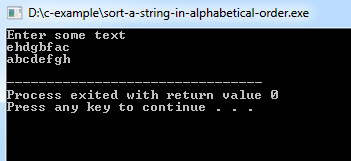## C Programming Examples Tutorial Index

This C program will show you how to sort a string in alphabetical order.

Program:

``````#include <stdio.h>
#include <stdlib.h>
#include <string.h>

void sort_string(char*);

int main()
{
char string;

printf("Enter some text\n");
gets(string);

sort_string(string);
printf("%s\n", string);

return 0;
}

void sort_string(char *s)
{
int c, d = 0, length;
char *pointer, *result, ch;

length = strlen(s);

result = (char*)malloc(length+1);

pointer = s;

for ( ch = 'a' ; ch <= 'z' ; ch++ )
{
for ( c = 0 ; c < length ; c++ )
{
if ( *pointer == ch )
{
*(result+d) = *pointer;
d++;
}
pointer++;
}
pointer = s;
}

*(result+d) = '\0';

strcpy(s, result);
free(result);
}``````

Program Output:Explanation:

This program will demonstrate you how to sort a string in the alphabet. So first of all, you have to include the stdio header file using the "include" preceding # which tells that the header file needs to be process before compilation, hence named preprocessor directive. Also, you have to include the string.h header file. The string.h header classifies one variable type, one macro, and various functions to manipulate arrays of characters within your program.

Then you have to define the main() function and it has been declared as an int as it is going to return an integer type value at the end of the program. Then inside main() you have to declare a character array name 'string' of size 100. Then you have to use printf() to display a message - "Enter some text". Then the gets() function will fetch the input string from the user.

Then the user-defined function sort_string() is called which is having 'string' as a parameter. Then the next printf() display the value of the string. Now inside the user-defined function, the definition will contain 3 integer type variables - c, d, length, and initialize d as 0. Then a pointer type character variable *pointer,  *result and a character variable 'ch'. The strlen() will count the length of the string and store the value in the form of integer to the variable length. result = (char*)malloc(length+1); statement will allocate memory for the variable 'result' and the value of 's' gets stored in 'pointer'.